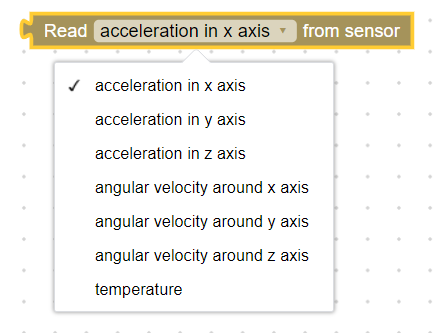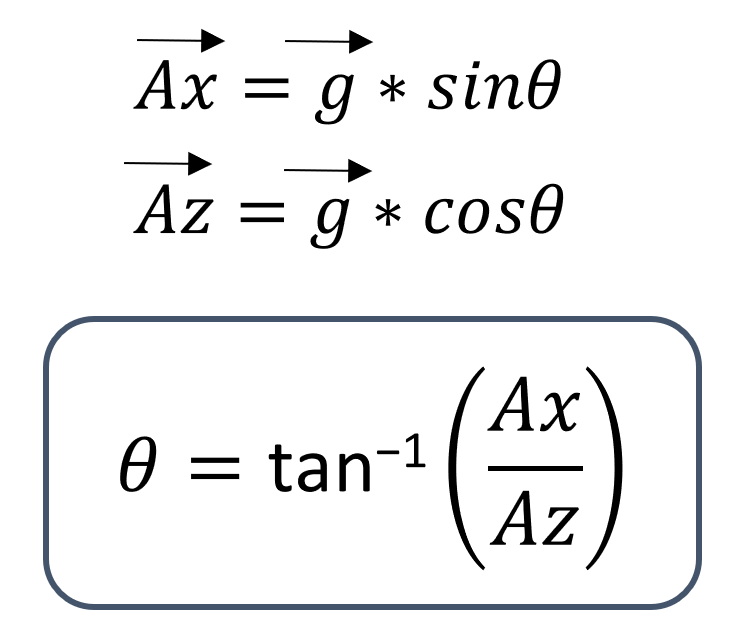top of page
Search

# Accelerometer

Updated: Feb 26, 2022

In this tutorial, we are going to learn how to use accelerometer in Nirmiti base shield. Nirmiti base shield has IMU (Inertial Motion unit) sensor , MPU6050.IMU parameters

## Block we are going to useThis block can be used to get acceleration along and angular velocity about all three axes X,Y and Z.

## How to measure angle using acceleration?

We know that earth has gravitiotional force which attract every object towards its center. So every steady object on earth has acceleration towards center of the earth though that object is not moving. This acceleration is called acceleration due to gravity(g) which is equal to 9.8m/s^2 .acceleration due to gravity

We are going to use this acceleration due to gravity for measuring the angle. Accelerometer measure acceleration in three axes. if sensor is facing upward then g (acceleration due to gravity) is along Z axis . If sensor is tilted , this g is divided along Z and other two axes X and Y. in following image you can see g is divided along Z and X axis.gravity vector and its componentsangle calulation from gravity

Above calculations are implemented in following example

## Exampleaccelerometer
.txt

Note : In above example , at the begining variable 'a' is assigned with -1 which tell that it can store positive and negative integers. Tf -1 was not assigned to 'a', 'a' would have store only positive numbers.